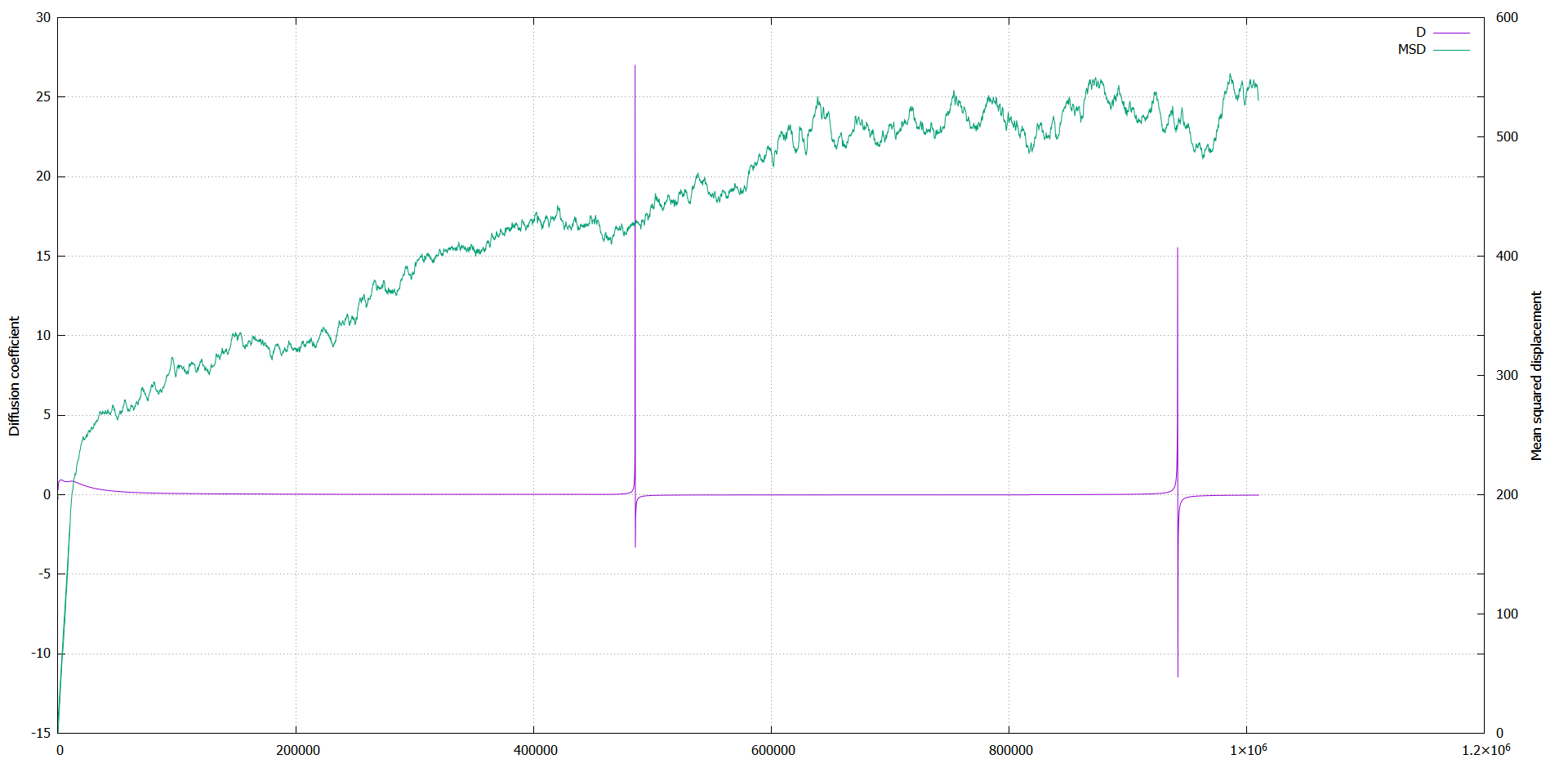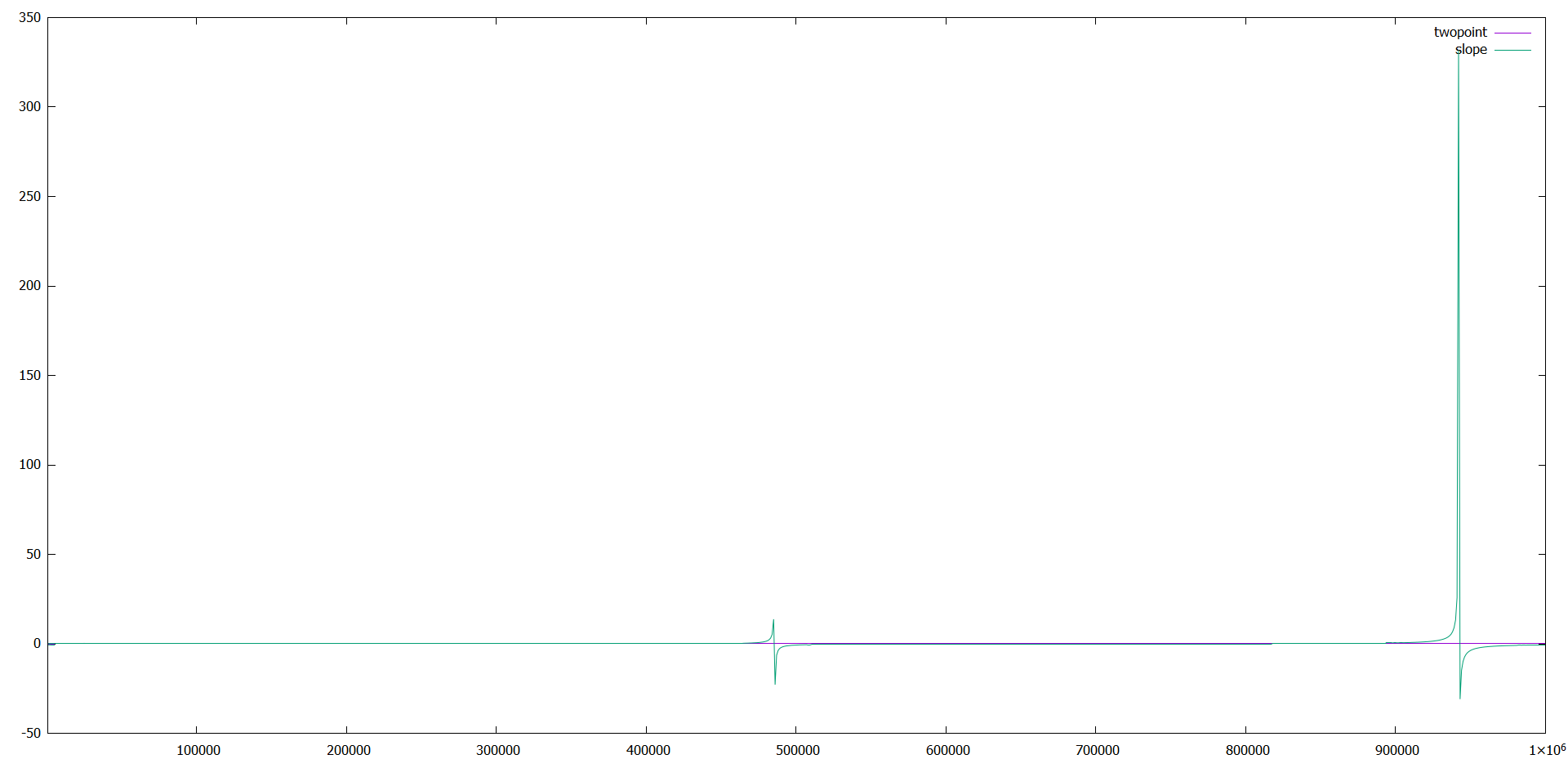# Weird diffusion coefficient

Dear all,

I simulate condensation gelation off colloid particles by use of Brownian dynamic.

I want to calculate the diffusion coefficient. To do so I use the msd method as in the example DIFFUSE.

In my input file it is as follows:

compute msd all msd

fix msd all vector 10 c_msd

variable diff equal slope(f_msd)/4/(10*dt)

variable test equal slope(f_msd)

First, everything seemed fine, but when I extended the simulations with more steps, problems appeared.

The diffusion coefficient shows unexpected behavior: it gets large peaks at certain timesteps, and after that it is negative.

Then it came back to a positive value, but at some moment again it peaks.

It does so for each simulation, independent of box size, amount of particles and size of timestep.

To see if it was a problem of my dynamics, I run the example in.msd.2d, except that I did a million steps instead of 100 K.

In the results, the same behavior appeared.

It seems to be a problem in slope(x), since the data of the msd is fine.

To check that, I plotted pure slope(f_msd), without dividing or multiplying it and indeed it gave the weird behavior.

I added a plot of my own data (MSD_D.png), and a plot of the example run for million steps.

Is this a bug or is there something I am missing? I use the LAMMPS version of 3Mar2020.

Any help would be appreciated.

M.J.